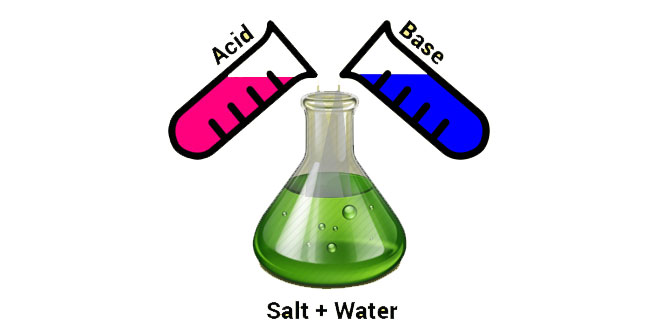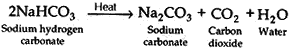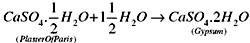Wednesday , May 18 2022# Acids, Bases and Salts: 10th Science

#### Question: Do basic solutions also have H+(aq) ions? If yes, why are these basic?

Answer: No, the basic solution doesn’t have H+ ions as the solution has excess of hydroxide ions.

#### Question: Under what soil condition do you think a farmer would treat the soil of his fields with quick lime (calcium oxide) or slaked lime (calcium hydroxide) or chalk (calcium carbonate)?

Answer: Most often the soil in the fields is too acidic. If the soil is too acidic (having low pH), it is treated with materials like quicklime (calcium oxide) or slaked lime (calcium hydroxide) or chalk (calcium carbonate). Thus, a farmer should add lime or slaked lime in his fields when the soil is too acidic.

#### Question: What is the common name of the compound CaOCl2?

Answer: The common name of the compound CaOCl2 is bleaching powder.

#### Question: Name the substance that on treatment with chlorine yields bleaching powder.

Answer: Calcium hydroxide is the substance that on treatment with chlorine yields bleaching powder.

#### Question: Name the sodium compound, which is used, for softening hard water.

Answer: Sodium carbonate (washing soda) is used for softening hard water.

#### Question: What will happen if a solution of sodium hydro carbonate is heated? Give the equation of the reaction involved.

Answer: Sodium hydrogen carbonate decomposes on heating forming sodium carbonate, carbon dioxide and water.#### Question: Write an equation to show the reaction between plaster of Paris and water.

Answer: Plaster of Paris has a very remarkable property of setting into a hard mass on wetting with water. So, when water is added to plaster of Paris, it sets into a hard mass in about half an hour. The setting of plaster of Paris is due to the hydration crystals of gypsum, which set to form a hard, solid mass.#### Question: Why does distilled water not conduct electricity, whereas rainwater does?

Answer: Distilled water does not conduct electricity because it does not contain any ionic compound (like acids, bases or salts) dissolved in it. On the other hand, rain water conducts electricity. This can be explained as follows: Rain water, while falling to the earth through the atmosphere, dissolves an acidic gas carbon dioxide from the air and forms carbonic acid (H2CO3). Carbonic acid provides hydrogen ions, H+(aq), and carbonate ions, CO2-3 (aq), to rain water. So, due to the presence of carbonic acid (which provides ions to rain water), the rain water conducts electricity.

#### Question: Why do acids not show acidic behavior in the absence of water?

Answer: The acidic behavior of acid is due to the presence of hydrogen ions. The acids will not show its acidic behavior in the absence of water, this is because the acids produce hydrogen ions only in the presence of water.

#### Question: Five solutions A, B, C, D and E when tested with universal indicator showed pH as 4, 1, 11, 7 and 9 respectively. Which solution is? (i) Neutral? (ii) Strongly alkaline? (iii) Strongly acidic? (iv) Weakly acidic? (v) Weakly alkaline? Arrange the pH in increasing order of hydrogen-ion concentration.

(i) The Solution D showing pH value of 7 is neutral.
(ii) The Solution C showing pH value of 11 is strongly alkaline.
(iii) The Solution B showing pH value of 1 is strongly acidic.
(iv) The Solution A showing pH value of 4 is weakly acidic.
(v) The Solution E showing pH value of 9 is weakly alkaline.

Increasing order of hydrogen ion concentration is: C < E < D < A < B

#### Question: Equal lengths of magnesium ribbons are taken in test tubes A and B. Hydrochloric acid (HCl) is added to test-tube A while acetic acid (CH3COOH) is added to test-tube B. In which test-tube will fizzing occur more vigorously and why?

Answer: Acetic acid (CH3COOH) is a weak acid whereas hydrochloric acid (HCl) is a strong acid. Fizzing occurs in the test tube due to the evolution of hydrogen gas by the action of acid on magnesium ribbon. Since hydrochloric acid is a strong acid a large amount of hydrogen gas is liberated in the test tube A. So fizzing occurs more vigorously in test tube A .

#### Question: Fresh milk has a pH of 6. How do you think the pH will change as it turns into curd? Explain.

Answer: The pH will change to below 6, as lactic acid is formed when milk turns into curd.

#### Question: Plaster of Paris should be stored in a moisture-proof container. Why?

Answer: The presence of moisture can affect the slow setting of plaster of Paris by bringing about its hydration. This will make the plaster of Paris useless after some time.

#### Question: What is a neutralization reaction? Give two examples.

Answer: The reaction of an acid and a base, giving rise to the corresponding salt and water is called neutralization reaction.

Generalized neutralization reaction can be written as:

Reaction of an acid and base to produce salt and water is known as neutralization reaction.
Example: HCL+ NaOH NaCL + H2O and HNO3 + KOH  KNO3 + H2O